International
Tables for
Crystallography
Volume C
Mathematical, physical and chemical tables
Edited by E. Prince

International Tables for Crystallography (2006). Vol. C, ch. 8.1, pp. 687-688

## Section 8.1.6. Orthogonal distance regression

E. Princea and P. T. Boggsb

aNIST Center for Neutron Research, National Institute of Standards and Technology, Gaithersburg, MD 20899, USA, and bScientific Computing Division, National Institute of Standards and Technology, Gaithersburg, MD 20899, USA

### 8.1.6. Orthogonal distance regression

| top | pdf |

It is often useful to consider the data for a least-squares problem to be in the form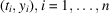, where theare considered to be the independent variables and thethe dependent variables. The implicit assumption in ordinary least squares is that the independent variables are known exactly. It sometimes occurs, however, that these independent variables also have errors associated with them that are significant with respect to the errors in the observations. In such cases, referred to as errors in variables' or measurement error models', the ordinary least-squares methodology is not appropriate and its use may give misleading results (see Fuller, 1987).

Let us define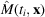to be the model functions that predict the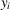. Observe that ordinary least squares minimizes the sum of the squares of the vertical distances from the observed pointsto the curve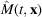. Ifhas an error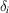, and these errors are normally distributed, then the maximum-likelihood estimate of the parameters is found by minimizing the sum of the squares of the weighted orthogonal distances from the pointto the curve. More precisely, the optimization problem to be solved is given by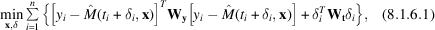where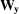and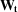are appropriately chosen weights. Problem (8.1.6.1)is called the orthogonal distance regression (ODR) problem. Problem (8.1.6.1)can be solved as a least-squares problem in the combined variables x, δ by the methods given above. This, however, is quite inefficient, since such a procedure would not exploit the special structure of the ODR problem. Few algorithms that exploit this structure exist; one has been given by Boggs, Byrd & Schnabel (1987), and the software, called ODRPACK, is by Boggs, Byrd, Donaldson & Schnabel (1989). The algorithm is based on the trust-region (Levenberg–Marquardt) method described above, but it exploits the special structure of (8.1.6.1)so that the cost of each iteration is no more expensive than the cost of a similar iteration of the corresponding ordinary least-squares problems. For a discussion of some of the statistical properties of the resulting estimates, including a procedure for the computation of the variance–covariance matrix, see Boggs & Rogers (1990).

### References

Boggs, P. T., Byrd, R. H., Donaldson, J. R. & Schnabel, R. B. (1989). ODRPACK – software for weighted orthogonal distance regression. ACM Trans. Math. Softw. 15, 348–364.
Boggs, P. T., Byrd, R. H. & Schnabel, R. B. (1987). A stable and efficient algorithm for nonlinear orthogonal distance regression. SIAM J. Sci. Stat. Comput. 8, 1052–1078.
Boggs, P. T. & Rogers, J. E. (1990). Orthogonal distance regression. Contemporary mathematics: statistical analysis of measurement error models and applications. Providence, RI: AMS.
Fuller, W. A. (1987). Measurement error models. New York: John Wiley & Sons.